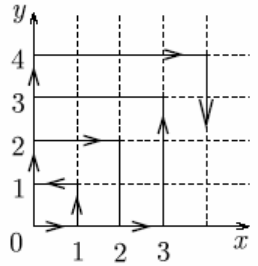Trang chủ / Toán Kangaroo / Lớp 7-8

USA Math Kangaroo Grade 7-8 in 2005

1. 2005 • 5002 =

2. How many hours are there in half of a third part of a quarter of a day?

3.

An ant is walking from point A to point B on a cube along the indicated path. The edge of the cube is 12 cm long. How far does the ant need to travel?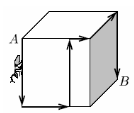4. The sum of the volume of three pitchers and two bottles equals 16 liters. The volume of each pitcher is two times greater than the volume of each bottle. The sum of the volume of two pitchers and three bottles is:

5. At our school, 50% of the students have bikes. Of the students who have bikes, 30% have skateboards. What percent of the students at our school have both a bike and a skateboard?

6. In triangle ABC, the measure of the angle at vertex A is three times the measure of the angle at vertex B and half the measure of the angle at vertex C. What is the measure of the angle at vertex A?

7. How many three-digit numbers are there in which all the digits are even?

8. The diagram shows the floor plan of a room. Adjacent walls are perpendicular to each other. Letters a and b represent the lengths of some the walls. What is the area of the room?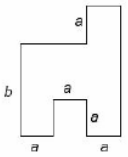9. In the diagram, the five circles have the same radii, and they touch as shown. The small square joins the centres of the four outer circles. The ratio of the shaded area of all the circles to the non-shaded area of all the circles is: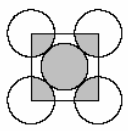10. There was a certain number of crows sitting on trees in the garden. If there had been just one crow sitting on each tree, then one crow would not have had a tree to sit on. However, if two crows had been sitting on each tree, then there would not be any crows on one tree. How many trees were there in the garden?

11. Problems 4 points each

Anna, Barbara, Teddy, and Wally went to a dance. They danced in pairs. Anna danced with Teddy and with Wally. Barbara danced with Teddy, but she didn’t dance with Wally. Decide which statement is false.

12. A group of classmates was planning a trip. If each of them paid \$14, then they would be \$4 short to pay for the trip. On the other hand, if each of them paid \$16, they would have \$6 more than they needed. How much should each of the classmates contribute so they collect the exact amount needed for the trip?

13. Carla cut a sheet of paper into 10 pieces. Then she took one piece and cut it again into 10 pieces. She then repeated this three more times. How many pieces of paper did she have after the last cutting?

14. A doorman works according the following schedule: he works for 4 consecutive days and has the fifth day off. Last Sunday he had the day off, and on Monday he started work according to his schedule. After how many days, including that Monday, will he have a day off on Sunday again?

15. Two rectangles ABCD and DBEF are shown in the picture.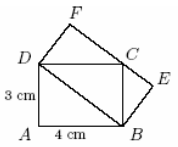What is the area of rectangle DBEF?

16. What is the measure of angle α indicated in the picture?

17. From noon until midnight, Clever Cat sleeps under the oak tree, and from midnight until noon he tells stories. There is a sign on the oak tree saying: “Two hours ago Clever Cat was doing the same thing that he will be doing in an hour.” How many hours a day is the information given on the sign true?

18. The diagram shows an equilateral triangle and a regular pentagon.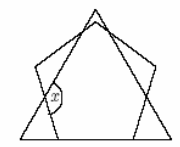What is the measure of angle x?

19. Which of the numbers below is the sum of four consecutive whole numbers?

20. Each pair vertices of a cube are connected with a segment. How many different points are there that are the midpoints of these segments?

21. Problems 5 points each

Let’s call the number of prime factors of a natural number n, the product of which is equal to the given natural number n, “the length”. For example, the length of 90 = 2x3x3x5 equals 4. How many odd numbers less than 100 have the length of 3?

22. In each of the four small squares shown in the picture, a different natural odd number less than 20 was written. Only one of the statements below is true.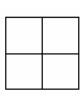Which one is it?

23. The sequence of letters AGKNORU in alphabetical order corresponds to a sequence of different digits placed in increasing order. What is the greatest number that corresponds with the word KANGOUROU?

24. Peter wrote down all three-digit numbers which have the following features: each number consists of three different digits, and the first digit is equal to the second power of the quotient of the second and the third digit. How many numbers did Peter write down?

25. Five lines: l1, l2, l3, l4, l5 intersect point O and they are intersected by five other lines k1, k2, k3, k4, k5 (see the picture).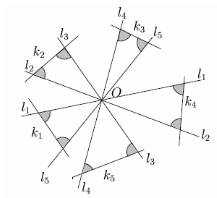What is the sum of the measures of 10 shaded angles, shown in the picture?

26. There were 64 liters of juice in a barrel. Then, 16 liters of juice were dumped out and replaced with 16 liters of water. After being mixed together, again 16 liters of the mixture were dumped and replaced with 16 liters of water. After being mixed together, this was done again, 16 liters of the mixture were dumped and replaced with water. How many liters of juice are there in the mixture now?

27. How many two-digit numbers with the ones digit greater than zero are there that are greater than three times the number that is created out of these numbers by reversing its digits?

28. ABC is a right triangle. AH is the height of the triangle and AK is a bisector of right angle A. If the ratio CK : KB equals 1/3 then the ratio CH : HB equals: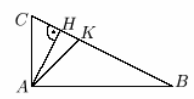29. If the average of 10 different positive integers is 10, the greatest of this numbers can be:

30. A particle moves through the first quadrant of the figure as follows: during the first minute it moves from the origin to (1,0). Then, it continues to follow the pattern indicated in the figure, going back and forth between the positive x and y axes, moving one unit of distance parallel to an axis in one minute. Which point will the particle reach after exactly 2 hours?